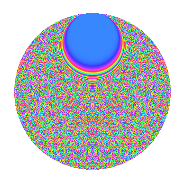# Properties

 Label 2541.2.caLevel 2541 Weight 2 Character orbit ca Rep. character $$\chi_{2541}(20,\cdot)$$ Character field $$\Q(\zeta_{110})$$ Dimension 13920 Sturm bound 704

# Related objects

## Defining parameters

 Level: $$N$$ = $$2541 = 3 \cdot 7 \cdot 11^{2}$$ Weight: $$k$$ = $$2$$ Character orbit: $$[\chi]$$ = 2541.ca (of order $$110$$ and degree $$40$$) Character conductor: $$\operatorname{cond}(\chi)$$ = $$2541$$ Character field: $$\Q(\zeta_{110})$$ Sturm bound: $$704$$

## Dimensions

The following table gives the dimensions of various subspaces of $$M_{2}(2541, [\chi])$$.

Total New Old
Modular forms 14240 14240 0
Cusp forms 13920 13920 0
Eisenstein series 320 320 0

## Trace form

 $$13920q - 508q^{4} - 86q^{7} - 54q^{9} + O(q^{10})$$ $$13920q - 508q^{4} - 86q^{7} - 54q^{9} + 204q^{16} - 134q^{18} - 77q^{21} - 52q^{22} + 180q^{25} - 96q^{28} - 178q^{30} + 14q^{36} - 172q^{37} - 88q^{39} + 29q^{42} - 176q^{43} - 212q^{46} - 154q^{49} - 148q^{51} - 68q^{57} + 8q^{58} - 110q^{60} - 66q^{63} - 300q^{64} - 112q^{67} - 206q^{70} - 350q^{72} - 18q^{78} - 108q^{79} - 126q^{81} - 47q^{84} - 316q^{85} - 64q^{88} - 130q^{91} - 284q^{93} - 356q^{99} + O(q^{100})$$

## Decomposition of $$S_{2}^{\mathrm{new}}(2541, [\chi])$$ into newform subspaces

The newforms in this space have not yet been added to the LMFDB.

## Hecke Characteristic Polynomials

There are no characteristic polynomials of Hecke operators in the database